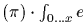Next: The Natural Logarithm Function Up: Mathematical Functions Previous: The Floor Function

### Gaussian Elimination

Calling Sequence:
GaussElim(
A, b)
Parameters:
 A : matrix(real) b : array(real)

Returns: a vector (one dimensional array) of real.

Synopsis: Given a matrix of real values A and vector b, this function computesby Gaussian elmination. A matrix is a two-dimensionsal square array.

Examples:

> GaussElim([[2,4,6], [9,0,27],[17,23,5]], [8, 15, 17]);


Gaston Gonnet
1998-09-15